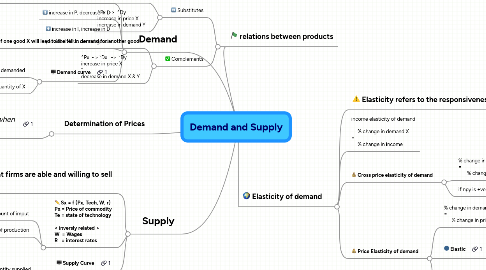# Demand and Supply

Topic 1 - Economics

Get Started. It's FreeDemand and Supply## 1. relations between products

### 1.1. Substitutes

1.1.1. Increase in the price of one good (X) will cause an increase in demand for another good (Y)

1.1.2. ^Px -> ^Dy increase in price X increase in demand Y

### 1.2. Complements

1.2.1. Increase in the price of one good X will lead to the fall in demand for another good

1.2.2. ^Px - > ˇDx -> ˇDy increase in price X ˇ decrease in demand X & Y

## 2. Demand

### 2.4. Demand curve

2.4.1. Negative relationship fall in price, increase in quantity demanded

2.4.1.1. increase in demand shifts the entire demand curve to the right

2.4.2. P against Quantity of X

## 3. Supply

### 3.2. Sx = f (Px, Tech, W, r) Px = Price of commodity Te = state of technology < inversly related > W = Wages R = interest rates

3.2.1. improvement in technology will cause more outputs to be produced with the same amount of imput

3.2.2. Wages and Interest Rates will lead to an increase in the cost of production

### 3.3. Supply Curve

3.3.1. Positive Relationship increase in prices will lead to an increase in quantity supplied

## 4. Determination of Prices

### 4.1. Equilibrum occurs when demand = supply

4.1.1. Above equilibrium price, P will fall

4.1.2. Below equilibrium price, P will rise

## 5. Elasticity of demand

### 5.3. Cross price elasticity of demand

5.3.1. % change in demand X = -------------------------- % change price Y

5.3.2. if npy is +ve, the good is a substitute

5.3.2.1. ^Py -> ^Dx

### 5.4. Price Elasticity of demand

5.4.1. % change in demand X = -------------------------- % change in price X

5.4.1.1. always -ve for a -ve slope demand curve

5.4.1.2. demand for a good is more sensitive when there is more substitutes in the market

5.4.2. Elastic

5.4.2.1. >1% change in demand per 1% change in price

5.4.2.2. perfectly elastic

5.4.3. Inelastic

5.4.3.1. <1% change in demand per 1% change in price

5.4.3.2. perfectly inelastic